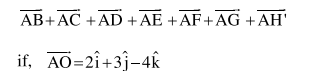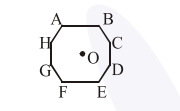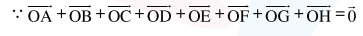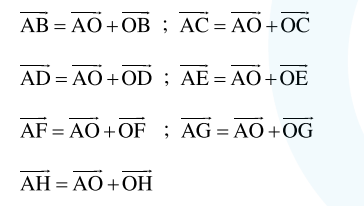# In an octagon A B C D E F G H of equal side, what is the sum of

Question:

In an octagon $A B C D E F G H$ of equal side, what is the sum of1. $-16 \hat{\mathrm{i}}-24 \hat{\mathrm{j}}+32 \hat{\mathrm{k}}$

2. $16 \hat{\mathrm{i}}+24 \hat{\mathrm{j}}-32 \hat{\mathrm{k}}$

3. $16 \hat{\mathrm{i}}+24 \hat{\mathrm{j}}+32 \hat{\mathrm{k}}$

4. $16 \hat{\mathrm{i}}-24 \hat{\mathrm{j}}+32 \hat{\mathrm{k}}$

Correct Option: , 2

Solution:

We know,By triangle law of vector addition, we can writeNow

$\overrightarrow{\mathrm{AB}}+\overrightarrow{\mathrm{AC}}+\overrightarrow{\mathrm{AD}}+\overrightarrow{\mathrm{AE}}+\overrightarrow{\mathrm{AF}}+\overrightarrow{\mathrm{AG}}+\overrightarrow{\mathrm{AH}}$

$=(7 \overrightarrow{\mathrm{AO}})+\overrightarrow{\mathrm{OB}}+\overrightarrow{\mathrm{OC}}+\overrightarrow{\mathrm{OD}}+\overrightarrow{\mathrm{OE}}+\overrightarrow{\mathrm{OF}}+\overrightarrow{\mathrm{OG}}+\overrightarrow{\mathrm{OH}}$

$=(7 \overrightarrow{\mathrm{AO}})+\overrightarrow{0}-\overrightarrow{\mathrm{OA}}$

$=(7 \overrightarrow{\mathrm{AO}})+\overrightarrow{\mathrm{AO}}$

$=8 \overrightarrow{\mathrm{AO}}=8(2 \hat{\mathrm{i}}+3 \hat{\mathrm{j}}-4 \hat{\mathrm{k}})$

$=16 \hat{\mathrm{i}}+24 \hat{\mathrm{j}}-32 \hat{\mathrm{k}}$